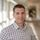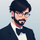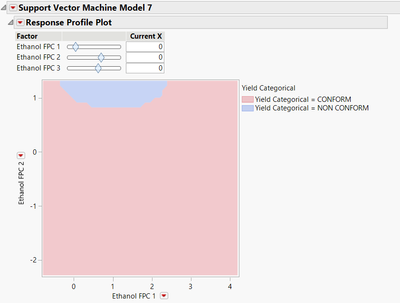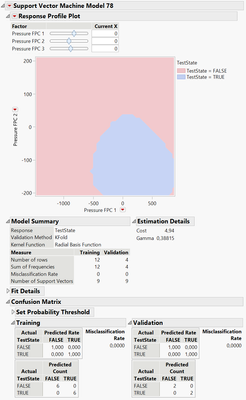Choose Language Hide Translation Bar

## Control Charting Kinetical and Other Time-Dependent Curves (2022-EU-30MP-1042)

SPC and control charting is a common procedure in industry. Normally, you are controlling and observing a single measure over time. These data are displayed with ±3s limits around the mean on a chart.

However, when kinetical curves or other time-dependent behaviors are a matter of quality and consistency of a process, it is much more difficult to display in a SPC chart. These curves are often displayed within their maximal and minimal specification for each time point, which makes the off-spec curves visible. How can “off-spec” curves be defined while staying within these max-min limits?

The first method to try might be the principal component analysis (PCA). If the runtime stamps are always the intervals, then it's easy to achieve results. However, if they vary, interpolation of the Ys that will be on the same stamp is needed, which complicates data preparation.

With the Functional Data Explorer in JMP Pro, it becomes very convenient to display the different curves as principal components in a T2-control chart.

This presentation shows how we used this tool for quality control for a pressure leakage test and how we made it simple for the practitioner to use.

Okay. Hello.

Thanks for the nice introduction.

Today I want to present together with Stefan Vinzens from LRE Medical

a work we've done in the last year on control charting kinetical

and other time- dependent measurements.

So why is that important to present?

So in case you have a time- dependent curves

and the curves themselves are important for the quality of your product,

so it's often very hard to define any kind of specification.

And in our case here,

these curves were evaluated by specialists.

So every measurement was sent to a specialist,

looked at the curve, said, "Yes, okay, or not okay."

This is pretty time- consuming and compassion and so on.

And on top of that, it's even bad when the person is sick or in vacation.

Also it's a person- dependent thing.

So often it happens that the person

has different moods or different obligation

or different priorities and so on.

So let's say the adjustment of the curve may vary a little bit.

So it's kind of reproducibility problem

And we wanted to stop that.

So that was the reason why we started with it.

So for example, here you see a selection from hundreds of these curves we measured.

And you see here it's a pressure holding measurement.

So we have here pressure versus time in seconds.

And here you see a bunch of curves.

And the green ones, you see they are called true.

This means true is accepted, they are good.

And false means rejected, they are not good.

So there is a bad product.

So what you see here that the green ones are relatively tied together.

Here in this highlighting screenshot, you see better.

So they're pretty close together.

And then we have a selection of red ones

which are either apart from the green ones,

but there are also two ones which are more or less in the same regime.

But as you see, they have completely different shapes.

There are some edges and other s-shaped curves and so on and to forth.

So if it would make just a simple t hree-sigma limit ±

around the good ones, for example, as in the upper case here,

we would include also the nongood red ones here from the lower picture.

So a simple ± three Sigma limit

approach around this series of curves would not be target leading.

So we need something which includes the position

which is done here with the basic monument.

But also we want to have something which takes care about the shape

of these functions, of these groups,

How to analyze the shapes and positions?

So there are actually two approaches.

One is pretty long- known already.

This is the principal component analysis.

That's the first one.

And in more recent times JMP came up with

a functional data explorer which also gives us the possibility to do the same.

But as we have seen, it was not really the same, so it was different.

For doing so, you need to transform the long table I just show you

in the next picture here on the left side,

a long table where you have columns

with the part number, for example, with the test date.

But also and this is the important part

of the runtime and the pressure and this for each value.

And you see here, this is in seconds.

So we measured every some milliseconds.

And as you see also, it is pretty hard that for the next part

it's exactly the same series of numbers here,

exactly the same time when we have the next pressure point.

And this is actually needed when you want

to make a principal component analysis because you have to transform

this long table into a wide table where you have one row per part

and then you have the white table

where you have for each time slot and then the data points.

So what we need to do now is, first of all,

we need to bring all these runtimes on the same scale.

Here, we have done that by just calculating

the longest time as 100% and the shortest is zero.

And then we have every numbers from millisecond seconds

transferred into this percent scale.

Then we interpolated because still they were not on the same time slot actually.

So we had to bring all the Y numbers on the same time point.

This means we need to do a kind

of interpolation to have them all on the same.

And then when we have that,

so we created a new column with the standard relative times.

I've just seen them in the example in the next slide, so 00001 and so on.

And then we transpose them into this white form.

And from there on, then we could do the principal component analysis,

saving the printable components and calculate the T squares from them

and build the control charts from these T squares values.

So here you see it's the slot 0%, 1%, 2% and so on.

And here are the pressure values.

In a parallel plot, it looks kind of like this.

So we have here the different slots here on the X- axis

and the pressure is still the same numbers as before.

And you see that the curves are looking pretty much the same as before.

But now we have about 100 data points and before we had thousands.

So it's the density of the points are a little less, but still the curves

and the shapes and the positions and everything are the same as before.

And we take now this white table

and on all these different time slots,

we do a principle component analysis.

Then we find here this scoreboard for it.

What you already see here is that in the middle part, we have here

all the green dots and the green ones, as you see here are the accepted ones

or the good parts surrounded by the red dots,

the rejected ones, the false ones.

And as you see also in this example here we can stay with two principal components

because the first principal components covers already 98% of the variation

in the second one, 2% and the other ones you can neglect.

So I think it's good enough to save just the first two principle components.

And then from these two principal components, we calculated the T squares.

This means T Square is principal component,

one squared plus principal components.

Two squares would be then the T squared here

for this data point.

Calculating this one .

So for every data point we calculated,

this T square and bring it on a control chart

Here, you see the control limits calculated only from the good,

from the green dots, from the good runs, from the good curve.

So you see it down here from the moving range.

So this means, okay. This controlling, it represents the normal natural variation,

what we expect from the good ones.

And all the non- good ones, the red ones

should be outside of this regime here.

And you see it's mostly done,

but not for these two points here for part number 14 and part number 15.

So they're inside the control limit.

But that is not what we want to see.

So first of all we have to understand what are these two.

So if you have a look onto the next picture,

then and highlight just these two, 14 and 15, then we see,

"Ah, okay." T hese are the ones here

which are really within the regime of the green ones.

The other ones which are further apart from it, they are easily excluded

or not excluded, but let's say distinguished

here with this control shot.

But these parts were not.

So what we learned from here, that is the principal component approach

as we have done it here or have done it in former times,

there is no information about the shape of the curve.

So we need something else which takes care about the shape of this curve.

So when we tested the FDE, the Function Data Explorer,

and the first good news is you can just take the long table as is.

So there's no need for data transformation

or bringing the different time points on the same slot number or whatever.

So you just take the raw data as they are.

Perhaps you will exclude some real outliers where there's the machine

has given wrong numbers or whatever.

But all the other stuff you can just take as is

and sometimes perhaps you don't want to do it on the transformation or so.

It's not really necessary.

And in this case, we have just taken the raw data as they are.

So starting doing an FDE on this,

we see here again our pressure time curve as we've seen them before.

But now you see these blue verticals here

and these blue verticals represent the number of knots.

So what is a knot?

So here we are fitting a spline curve.

And the spline curve is, let's say if you take a ruler

and then you want to make it a bit more flexible

to bend it onto all these different curves

or, let's say, to adjust it the best way to the data.

And the more, not this ruler has the more flexible it is.

And so the better you can adjust all these different points.

Here, we used these 20 knots thing.

So these are 20 verticals here and here with the basic information criterion,

you see that we get on all different functions.

We get the smallest BICs.

And just have an optical control on this,

you see on the lower left, all the data points separated out

for all the different curves here and with a red line on top of it

representing the spline curve which we fitted.

It doesn't matter if the curve is pretty straightforward

like here or has more edges or whatever,

it's perfectly aligned.

So just optically, it looks very good.

And you can also check that with the diagnostic plots.

For example, here this blind curve,

the predicted values are displayed versus the actual ones.

And you see they are perfectly aligned around these 45 degree line here.

And also the residuals, the ones are left and right here

from this prediction line, they are pretty small,

so there's not a lot of error left, which is not represented by this blanker.

So the fit looks really, really excellent.

So we have not taking the parameters for this split,

sorry for the spline and did a functional FDE.

Well, I'm completely confused today.

And we did a principal component analysis on these.

So we separated the eigenvalues,

which is the weighing factors, and the eigen functions

for each of these curves.

Then we can display them on a scoreboard.

Here the eigenvalues for each of these curves.

And you see here,

again with the BIC credit criterion,

you see how many numbers of functional principle

components you should optimally use.

And you see is the minimum with two.

So we stayed with two again as the one before.

And now we have this score board,

and it looks pretty much the same as the one before.

We have here the center part, the green of the curves, which are good,

surrounded by the red ones, which are rejected from the specialist.

And now we saved all these functional prints

and components and built a T square plot on it.

And here again, the same picture, the square.

If we take all the data points

to calculate the control limits, okay, then besides these two, everything

is part is in control.

But this is actually not what we want to have.

So we want to understand what is the normal variation of the good ones

to differentiate them from the variation of the non- good ones.

And so down here on the second plot here, we calculated the control limits

only from the good ones.

And then you see, okay, nearly all red curves are outside.

We're out of control. But there are two.

So the number 14 and the number two here are again directly on the borderline.

But all the others are clearly separated.

So let's have a look on the two and 14 which curves these are.

And then we see here. Ah, okay.

So they are really different from the red ones, that's clear.

But also kind of different from the majority of the green ones.

So the number two, which is defined as being true is the one

with a steeper slope here compared to all the other green ones here.

And the number 14 is this guy here,

which is more or less on the upper end of the regime of the greens.

So obviously, this little differences here in the shape is not

strong enough to come up really in this statistical calculation.

But we could really exclude here this guy here

from the others with a strong edge here.

And perhaps this one if the expert would have a look onto this

second time or perhaps it would

have rejected it because it's too steep a slope or whatever.

So these are border lining, I would say in both cases here

with the statistical approach but also, I guess with the manual approach.

But we could clearly detect number 15, which is the one here in the middle,

as being a non- normal behavior with this tool.

So you see, the FDE has also some limitations.

But overall, in addition to the standard PCA approach,

it comes up with these shape information of the curves.

And this is also part of distinguishing in a control chart

if a curve or a measurement is normally varying or is it not normal anymore.

To conclude these things, the standard PCA approach combined

with the T square analysis or control chart

is good for detecting the position of the curve

and distinguish them from curves which are not in this regime.

But as we have seen, it's lacking if the shape of the curve is of importance.

And with the FDE approach, again, combined with the T square analysis,

first of all, it needs much, much, much a less startup preparation.

But on top of that, it's good for checking the position as the above,

but also because it has shape information of the curves.

It is also including that in the good nongood understanding.

In our work, we stopped at this point,

but principally, you can build a kind of automatic.

If you use these curves or these principal components

and the information of good and bad, you can automize the good and bad.

But if you have a model behind which predicts you,

then what you will see.

But we haven't done that.

We stopped here on the T square chart to understand

the variation and what's varying more than normal variation.

Thank you very much for your attention.

And if you have questions, I'm open to answer them now.

Thank you.I'm having issues playing the video on this page. Is there something wrong with the file or link?@msulzer_bsi thank you for letting us know of the issue. The issue appears to be related to the translation service. You can view the video by following these steps:

1. Click the Choose Language link above the article2. Click View Originally Published Version

This should allow you to view the video. If you find this does not solve the problem, please let me know. In the meantime, we will investigate a fix. Thank you.@markus: very nice and interesting presentation. Have you considered that approach for spectral data? The issue of identifiying an  identiy of a material, in regard to a standard, as part of a standard QC inspection crossed my mind.Danke, SHS!
Auf diesem Weg habe ich es mit Spektraldaten noch nicht gemacht. Ich habe sie direkt in die Hauptkomponenten zerlegt und dann einem Kontrollchart zugeführt. Der Vorteil beim Functional Data Explorer ist, dass die Daten für die verschiedenen Runs nicht alle auf dem gleichen Zeit-Slot liegen müssen. Bei Spektraldaten ist das normalerweise kein Problem. Ich denke, dass es prinzipiell auch mit Spektren gehen sollte.

Grüße

MarkusHallo Markus,

Maybe also another option to try for this topic would be to use SVM for the analysis (if you have enough data), as it would be perhaps more "sensitive" ?
The process in this case would be :
1) Use Functional Data Explorer and model the curves.
2) Create the FPCs and export the table.
3) Use FPCs as factors and categorical Conform/Non-conform assessment as your response for the SVM model.

Just for illustration, you would be able to have a mapping of your response depending on the FPCs like this (example on "fermentation process" in JMP use cases) :I don't know if it would work on your example, but it could be an interesting alternative option.
Looking forward to hearing your thoughts on this,

VictorHi Victor

With my limited dataset from the presentation I found a good misclassification matrix using the SVM. You are right, it might be a possibility for these kind of data, too. I will test with the larger dataset next.Thanks for the hint!

Markus

Filter By
Article Tags
Contributors